Navigation

Test signals

Along with listening tests SoundExpert performs objective measurements of audio devices and technologies. For the purpose a new audio metric is used. The core of the method is testing with some input signals and measuring level of their waveform degradation at the output. While test signals can be of any kind the measurement procedure stays the same and waveform degradation is measured with a single parameter - Difference level (dB). Below is a list of actual reference signals along with their time domain representation, frequency domain representation and Matlab code for their generation. All signals are 30s long, -10dBFS(rms), stereo, 16bit and have 44100Hz sample rate.

Sine wave 1 kHz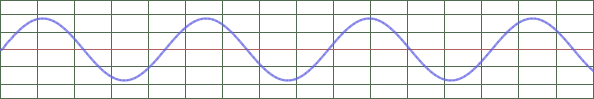% Sin wave 1000 Hz
% 30s, -10 dBFS (rms), Stereo (L=R), 44100 Hz, 16 bit
Fs = 44100; % [Hz]
L = 30; % [s]
G = -10; % [dB]
F = 1000; % [Hz]
Ls = round(L*Fs);
t = (0:Ls-1)';
ref = 10^(G/20) * sin(t*2*pi/(Fs/F));
ref = ref .* (2^15);
ref = round(ref);
ref = [ref,ref];
audiowrite('ref_sine1k.wav', int16(ref), Fs, 'BitsPerSample',16)

Sine wave 12.5 kHz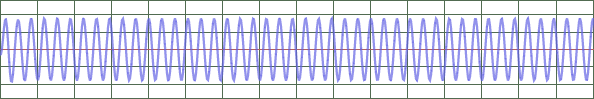% Sin wave 12500 Hz
% 30s, -10 dBFS (rms), Stereo (L=R), 44100 Hz, 16 bit
Fs = 44100; % [Hz]
L = 30; % [s]
G = -10; % [dB]
F = 12500; % [Hz]
Ls = round(L*Fs);
t = (0:Ls-1)';
ref = 10^(G/20) * sin(t*2*pi/(Fs/F));
ref = ref .* (2^15);
ref = round(ref);
ref = [ref,ref];
audiowrite('ref_sine12.5k.wav', int16(ref), Fs, 'BitsPerSample',16)

DFD 12.5 kHz / 80 Hz

Difference Frequency Distortion (DFD) signal is described in the standards IEC60118 and IEC60268. It consists of two pure tones: 12460 Hz and 12540 Hz and is useful for assessment of intermodulation distortion.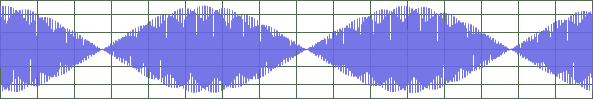% DFD 12.5 kHz mean, 80 Hz difference (12460 Hz + 12540 Hz)
% 30s, -10 dBFS (rms), Stereo (L=R), 44100 Hz, 16 bit
Fs = 44100; % [Hz]
L = 30; % [sec]
PdB = -10; % [dB]
F1 = 12460; % [Hz]
F2 = 12540; % [Hz]
Ls = round(L*Fs);
t = (0:Ls-1)';
ref = sin(t*2*pi/(Fs/F1)) + sin(t*2*pi/(Fs/F2));
Pr = sqrt(mean(ref.^2));
Pd = 10^(PdB/20)/sqrt(2);
Kp = Pd/Pr;
ref = ref .* Kp;
ref = ref .* (2^15);
ref = round(ref);
ref = [ref,ref];
audiowrite('ref_dfd12.5k.wav', int16(ref), Fs, 'BitsPerSample',16)

Square wave 1 kHz

Band-limited version of square wave is used. It is generated by means of Fourier synthesis (http://www.dspguide.com/ch13/4.htm):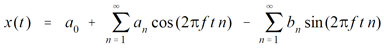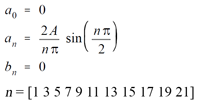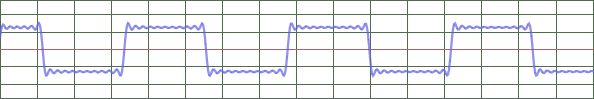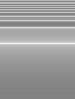% Fourier synthesis of square wave 1000 Hz
% 30s, -10 dBFS (rms), Stereo (L=R), 44100 Hz, 16 bit
Fs = 44100; % [Hz]
L = 30; % [sec]
PdB = -10; % [dB]
F = 1000; % [Hz]
Ls = round(L*Fs);
t = (0:Ls-1)';
n = [1 3 5 7 9 11 13 15 17 19 21]; % harmonics for 44100Hz sample rate only
A0 = 0;
Bn = 0;
ref = zeros(Ls,1) + A0;
for N = n
An = sin(pi*N/2) * 2 / (pi*N);
ref = ref + An*cos(t*2*pi*N/(Fs/F)) - Bn*sin(t*2*pi*N/(Fs/F));
end
Pr = sqrt(mean(ref.^2));
Pd = 10^(PdB/20)/sqrt(2);
Kp = Pd/Pr;
ref = ref .* Kp;
ref = ref .* (2^15);
ref = round(ref);
ref = [ref,ref];
audiowrite('ref_square1k.wav', int16(ref), Fs, 'BitsPerSample',16)

Triangle (odd) wave 1 kHz

Band-limited version of triangle wave is used. It is generated by means of Fourier synthesis (http://www.dspguide.com/ch13/4.htm):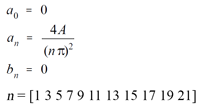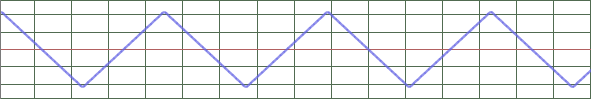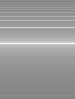% Fourier synthesis of triangle wave 1000 Hz
% 30s, -10 dBFS (rms), Stereo (L=R), 44100 Hz, 16 bit
Fs = 44100; % [Hz]
L = 30; % [sec]
PdB = -10; % [dB]
F = 1000; % [Hz]
Ls = round(L*Fs);
t = (0:Ls-1)';
n = [1 3 5 7 9 11 13 15 17 19 21]; % harmonics for 44100Hz sample rate only
A0 = 0;
Bn = 0;
ref = zeros(Ls,1) + A0;
for N = n
An = 4 / (N*pi)^2;
ref = ref + An*cos(t*2*pi*N/(Fs/F)) - Bn*sin(t*2*pi*N/(Fs/F));
end
Pr = sqrt(mean(ref.^2));
Pd = 10^(PdB/20)/sqrt(2);
Kp = Pd/Pr;
ref = ref .* Kp;
ref = ref .* (2^15);
ref = round(ref);
ref = [ref,ref];
audiowrite('ref_triangle(odd)1k.wav', int16(ref), Fs, 'BitsPerSample',16)

Triangle (even) wave 1 kHz

This is an even-only harmonics companion of standard triangle signal. It is generated by means of Fourier synthesis (http://www.dspguide.com/ch13/4.htm) like triangle signal but using even-only set of harmonics :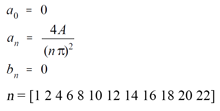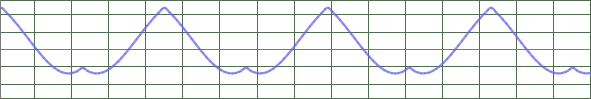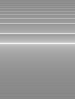% Fourier synthesis of triangle (even) wave 1000 Hz (ass train)
% 30s, -10 dBFS (rms), Stereo (L=R), 44100 Hz, 16 bit
Fs = 44100; % [Hz]
L = 30; % [sec]
PdB = -10; % [dB]
F = 1000; % [Hz]
Ls = round(L*Fs);
t = (0:Ls-1)';
n = [1 2 4 6 8 10 12 14 16 18 20 22]; % harmonics for 44100Hz sample rate only
A0 = 0;
Bn = 0;
ref = zeros(Ls,1) + A0;
for N = n
An = 4 / (N*pi)^2;
ref = ref + An*cos(t*2*pi*N/(Fs/F)) - Bn*sin(t*2*pi*N/(Fs/F));
end
Pr = sqrt(mean(ref.^2));
Pd = 10^(PdB/20)/sqrt(2);
Kp = Pd/Pr;
ref = ref .* Kp;
ref = ref .* (2^15);
ref = round(ref);
ref = [ref,ref];
audiowrite('ref_triangle(even)1k.wav', int16(ref), Fs, 'BitsPerSample',16)

White noise

Band-limited White Noise (20Hz-20kHz)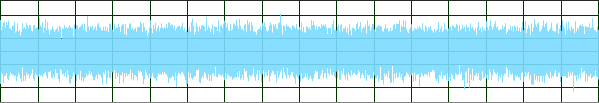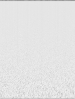% Band-limited White Noise (20Hz-20kHz)
% -10 dBFS RMS, 30sec, Stereo (L=R)
Fs = 44100; % [Hz]
PdB = -10; % RMS
Fss = 176400; % sampling freq [Hz]
T = 30; % length of signal [sec]
f1 = 20; % lowest freq [Hz]
f2 = 20000; % highest freq [Hz]
load ph_se-wn2020-crest9dB.mat; % phases for the crest factor = 9dB
a = ones(1,length(ph)); % amplitudes
fftd = zeros(1,Fss*T,'like',1+1i);
Z = a .* exp(1i .* ph);
Zc = fliplr(conj(Z));
fftd(T*f1+1 : T*f2+1) = Z;
fftd(end-T*f2+1 : end-T*f1+1) = Zc;
sig = ifft(fftd,'symmetric');
sig = sig(1:4:end); % sample from Fss for 44.1kHz
Ps = sqrt(mean(sig.^2));
Pd = 10^(PdB/20)/sqrt(2);
sig = round(sig.*(2^15)*Pd/Ps);
sig = [sig; sig]';
audiowrite('ref_bl-whitenoise.wav', int16(sig), Fs, 'BitsPerSample',16)

IEC 60268-1 (pink) noise (20Hz-20kHz)

A special test signal "Program Simulation Noise", whose spectral content is representative of music and speech (defined in the standard IEC 60268-1).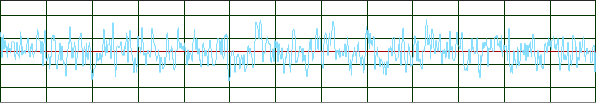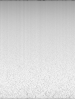% Band-limited IEC 60268-1 (pink) noise (20Hz-20kHz)
% -10 dBFS RMS, 30sec, Stereo (L=R)
Fs = 44100; % [Hz]
PdB = -10; % rms
Fss = 176400; % sampling freq [Hz]
T = 30; % length of signal [sec]
f1 = 20; % lowest freq [Hz]
f2 = 20000; % highest freq [Hz]
f = f1:1/T:f2; % vector of freqs
% powers in 1/3 octave bands according to IEC 60268-1
f268 = [20 25 31.5 40 50 63 80 100 125 160 200 250 315 400 500 630 800 1000 ...
1250 1600 2000 2500 3150 4000 5000 6300 8000 10000 12500 16000 20000];
a268 = [-13.4 -10.2 -7.4 -5.2 -3.5 -2.3 -1.4 -0.9 -0.5 -0.2 -0.1 0 0 0 0 0 ...
0 -0.1 -0.3 -0.6 -1 -1.6 -2.5 -3.7 -5.1 -7 -9.4 -11.9 -14.8 -18.2 -21.6];
a = interp1(f268, a268, f, 'linear', 0);
a = 10 .^ (a ./ 10); % vector of 1/3 octave powers
a = a ./ f; % vector of FFT powers (thanks to Alex)
a = sqrt(a); % vector of FFT amplitudes
% load ph_se-psn2020-CF6.mat; % phases for the crest factor = 6dB
load ph_se-psn2020-CF9.mat; % phases for the crest factor = 9dB
% load ph_se-psn2020-CF12.mat; % phases for the crest factor = 12dB (will
% clip if target RMS level of the signal > -12dB)
fftd = zeros(1,Fss*T,'like',1+1i);
Z = a .* exp(1i .* ph);
Zc = fliplr(conj(Z));
fftd(T*f1+1 : T*f2+1) = Z;
fftd(end-T*f2+1 : end-T*f1+1) = Zc;
sig = ifft(fftd,'symmetric');
sig = sig(1:4:end); % sample from Fss for 44.1kHz
sigPN = sig; % for the next signal
Ps = sqrt(mean(sig.^2));
Pd = 10^(PdB/20)/sqrt(2);
sig = round(sig.*(2^15)*Pd/Ps);
sig = [sig; sig]';
audiowrite('ref_iec60268-1.wav', int16(sig), Fs, 'BitsPerSample',16)

IEC 60268-1 (pink) noise, 1 bit

Program Simulation Noise downscaled to 1 bit (-101.1 dBFS RMS)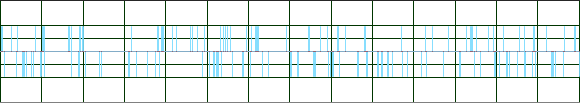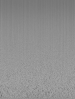% Band-limited IEC 60268-1 (pink) noise, 1bit(-101,1 dBFS RMS)
% 30sec, Stereo (L=R)
% "sigPN" is from previous signal - Band-limited IEC 60268-1 (pink) noise (20Hz-20kHz)
sig = sigPN ./ max(abs(sigPN));
sig = round(sig); % quantize
sig = [sig; sig]';
audiowrite('ref_iec60268-1_1bit.wav', int16(sig), Fs, 'BitsPerSample',16)

MOD-SMPTE 4:1 (60Hz/7kHz)

The standard signal that consists of two pure tones: 60 Hz and 7000 Hz and is useful for measurement of intermodulation distortion.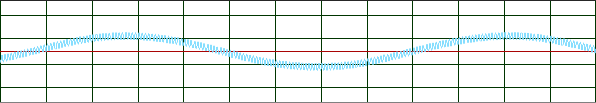% MOD-SMPTE 4:1 (60 Hz + 7 kHz, 4:1)
% -10 dBFS (rms), 30sec, Stereo(L=R)
Fs = 44100; % [Hz]

L = 30; % [sec]
PdB = -10; % [dB]
F1 = 60; % [Hz]
F2 = 7000; % [Hz]
Ls = round(L*Fs);
t = (0:Ls-1)';
out = 4 .* sin(t*2*pi/(Fs/F1)) + sin(t*2*pi/(Fs/F2));
Po = sqrt(mean(out.^2));
Pd = 10^(PdB/20)/sqrt(2);
Kp = Pd/Po;
out = out .* Kp;
% Scale to 16bit limits and quantize
out = out .* (2^15);
out = round(out);
out = [out,out];
audiowrite('ref_modsmpte41.wav', int16(out), Fs, 'BitsPerSample',16)

BS EN 50332-1

A special test signal "Program Simulation Noise", whose spectral content is representative of music and speech. It can be created by passing pink noise through a special filter network defined in IEC 60268-1. BS EN 50332 adds an additional requirement - that the crest factor of the test signal (the ratio between the instantaneous peak level of the signal and its RMS level) be between 1.80 and 2.2. Here it is generated with APx Waveform Generator Utility v4.2. The signal is used for setting volume level of DUT to EU loudness limit (EN 50332-2).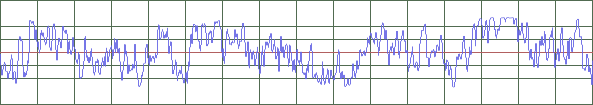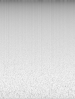% Pink Noise (BS EN 50332-1)
% 30s, -10 dBFS (rms), Stereo (L=R), 44100 Hz, 16 bit
Fs = 44100; % [Hz]

PdB = -10; % [dB]
ref = double(ref);
Pr = sqrt(mean(ref.^2));
Pd = 10^(PdB/20)/sqrt(2);
Kp = Pd/Pr;
ref = ref .* Kp;
ref = ref .* (2^15);
ref = round(ref);
ref = [ref,ref];
audiowrite('se_bsen50332-1-10dBFS.wav', int16(ref), Fs, 'BitsPerSample',16)

Test set of music material "Variety"

The new audio metric allows to measure waveform degradation using music as a test signal. The following 35 tracks of various genres are used for objective measurements of audio equipment. You can see results of such measurements in the form of histogram on Portable players page and in the form of diffrogram (for each track) in corresponding articles.

#. (year) Album - Artist - Track name

1. (1932) Russian Religion Chants - Theodore Chaliapine - Twofold Litany. Glory to Thee, O Lord
2. (1959) Kind Of Blue - Miles Davis - Blue In Green
3. (1960) Elvis Is Back! - Elvis Presley - Fever
4. (1962) The Nutcracker (Complete Ballet) - LSO, Antal Dorati - Act II - Waltz Finale and...
5. (1967) Sgt. Pepper's Lonely Hearts Club Band - The Beatles - A Day in the Life
6. (1968) Ogdens' Nut Gone Flake - The Small Faces - Ogdens' Nut Gone Flake
7. (1971) Hunky Dory - David Bowie - Oh! You Pretty Things
8. (1975) In Los Angeles - James Last - Bolero '75
9. (1977) Exodus - Bob Marley + Wailers - Jamming
10. (1977) I Remember Yesterday - Donna Summer - I Feel Love
11. (1977) Marquee Moon - Television - See No Evil
12. (1981) Nightclubbing - Grace Jones - I've Done It Again
13. (1982) Thriller - Michael Jackson - Beat It
14. (1983) Swordfishtrombones - Tom Waits - Frank's Wild Years
15. (1984) The Pearl - Harold Budd & Brian Eno - The Pearl
16. (1986) Graceland - Paul Simon - Under African Skies
17. (1987) Solitude Standing - Suzanne Vega - Tom's Diner
18. (1990) Violator - Depeche Mode - Halo
19. (1993) Bach Oratorios & Contatas - Collegium Vocale, Ph.Herreweghe - Choral. Der Lebensfurst, Herr Jesu Christ (BWV 43)
20. (1994-96) Alfred Schnittke. The Complete String Quartets - Kronos Quartet - String Quartet No. 4 - IV. Vivace
21. (1994) Grace - Jeff Buckley - Corpus Christi Carol
22. (1996) Richard D. James Album - Aphex Twin - Logan Rock Witch
23. (1996) Unchained - Johnny Cash - Rusty Cage
24. (1997) Homogenic - Bjork - Hunter
25. (1997) OK Computer - Radiohead - Exit Music (For a Film)
26. (2000) Cult - Apocalyptica - Fight Fire With Fire
27. (2000) Tourist - St. Germain - What You Think About...
28. (2003) Tour De France - Kraftwerk - Chrono
29. (2004) Amassakoul - Tinariwen - Amidinin
30. (2004) Careless Love - Madeleine Peyroux - J'ai Deux Amours
31. (2005) Takk... - Sigur Rós - Með Blóðnasir
32. (2007) The Trentemoller Chronicles - Trentemoller - Klodsmajor
33. (2008) This and That - Benjamin Grosvenor - Concert Study No.3 - Toccatina
34. (2013) Psychic - Darkside - Paper Trails
35. (2015) To Pimp a Butterfly - Kendrick Lamar - For Free (Interlude)